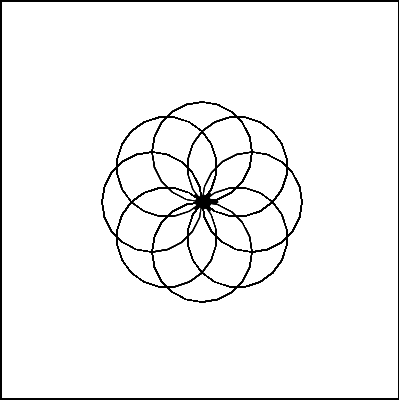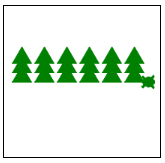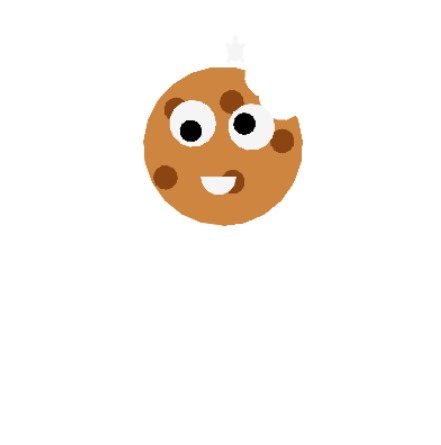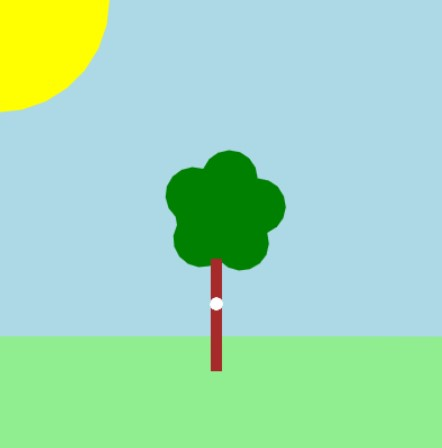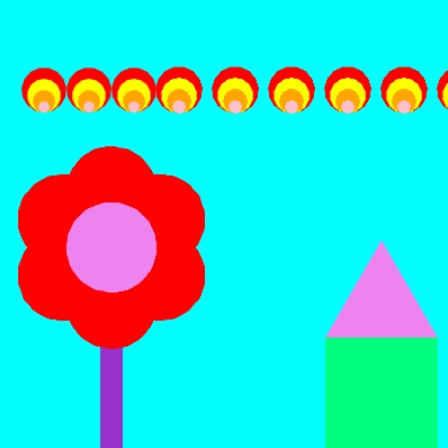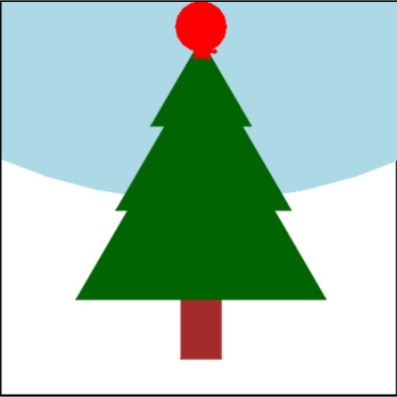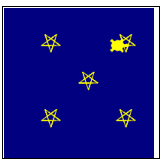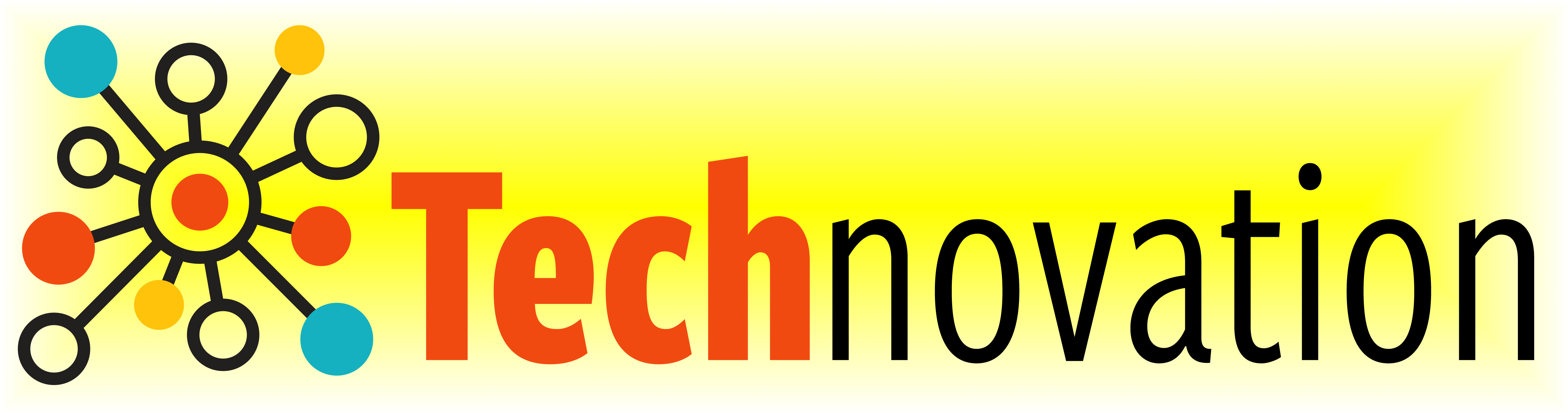# 7.4. Practice Makes Perfect¶

Here’s a reminder of the drawing commands we’ve learned so far:

Drawing commands

What does it do?

`forward( distance )`

Move forward a specified distance

`backward( distance )`

Move backward a specified distance

`left(90)`

Turns 90 degrees to the left (you can use any angle, not just 90!)

`right(90)`

Turns 90 degrees to the right

`circle( radius )`

Draws a circle with the specified radius

`goto( x, y )`

Move straight to the position with coordinates (x, y). Note: the center is (0, 0)

`up()`

Stop leaving a trail

`down()`

Start drawing a trail

`color( c )`

Set the color to c (https://trinket.io/docs/colors)

`begin_fill()`

Starts filling in drawn shapes

`end_fill()`

Stops filling in drawn shapes

`speed(number 0-10)`

Determines how quickly the turtle will move through commands

Functions and Loops

Drawing commands

What does it do?

`for i in range( n ):`

Repeat n times

`def function_name():`

Creates and defines a new function

`function_name()`

Calls a function

Variables

Command

What does it do?

`variable = value`

Stores the value in your variable

`variable = input( message )`

Takes user input and stores it in your variable

## 7.4.1. Quick review of variables:¶

• A variable is a container that stores a value

• You can access that value by calling your variable by its name

• You can change the value that’s stored in a variable with the equal sign “=”

Take a look at the example below that draws 3 circles in a row that are the same size without a variable versus with a variable.

Building off of this idea, can we draw a square that uses a variable for the length of its sides?

## 7.4.2. Parameters¶

Parameters allow us to use variables to make functions more customizable. When we call `turtle.circle(50)` we give it a value for the radius of the circle. We can do this when we define our own functions too.

Let’s try it out by writing a function that draws a square with a side length of our choice. Use your code from the previous exercise as a starting point.
Once you’ve got the above code working, try adding a second parameter that sets the color of the square!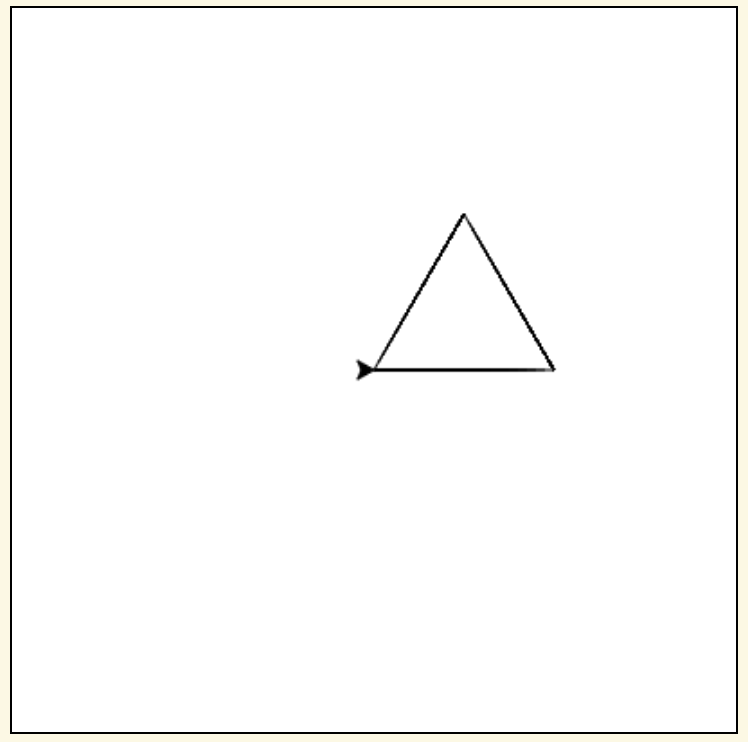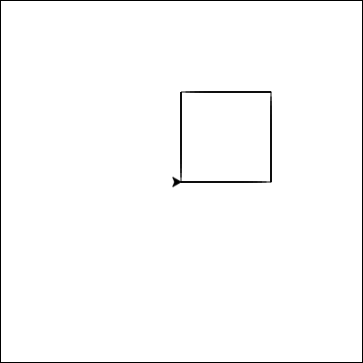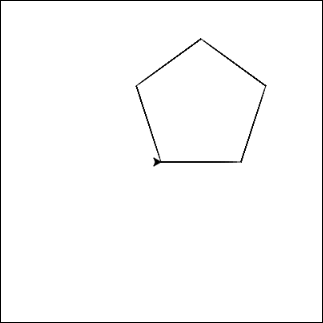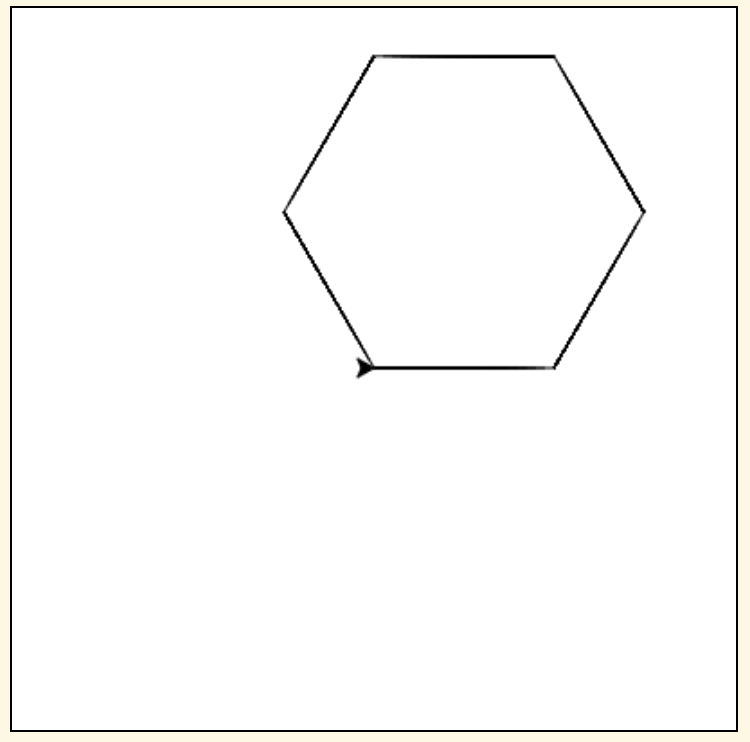How else can we use parameters? Let’s define a `shape()` function that has a parameter for the number of sides for the shape. (So `shape(3)` draws a triangle, `shape(4)` draws a square, etc)

## 7.4.3. Have Some Fun!¶

With what time we have left, take what you’ve learned and have some fun experimenting with drawing something cool! If you need some inspiration, check out the examples below! And, as always, ask a mentor if you get stuck or need any tips!